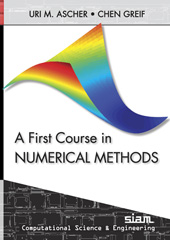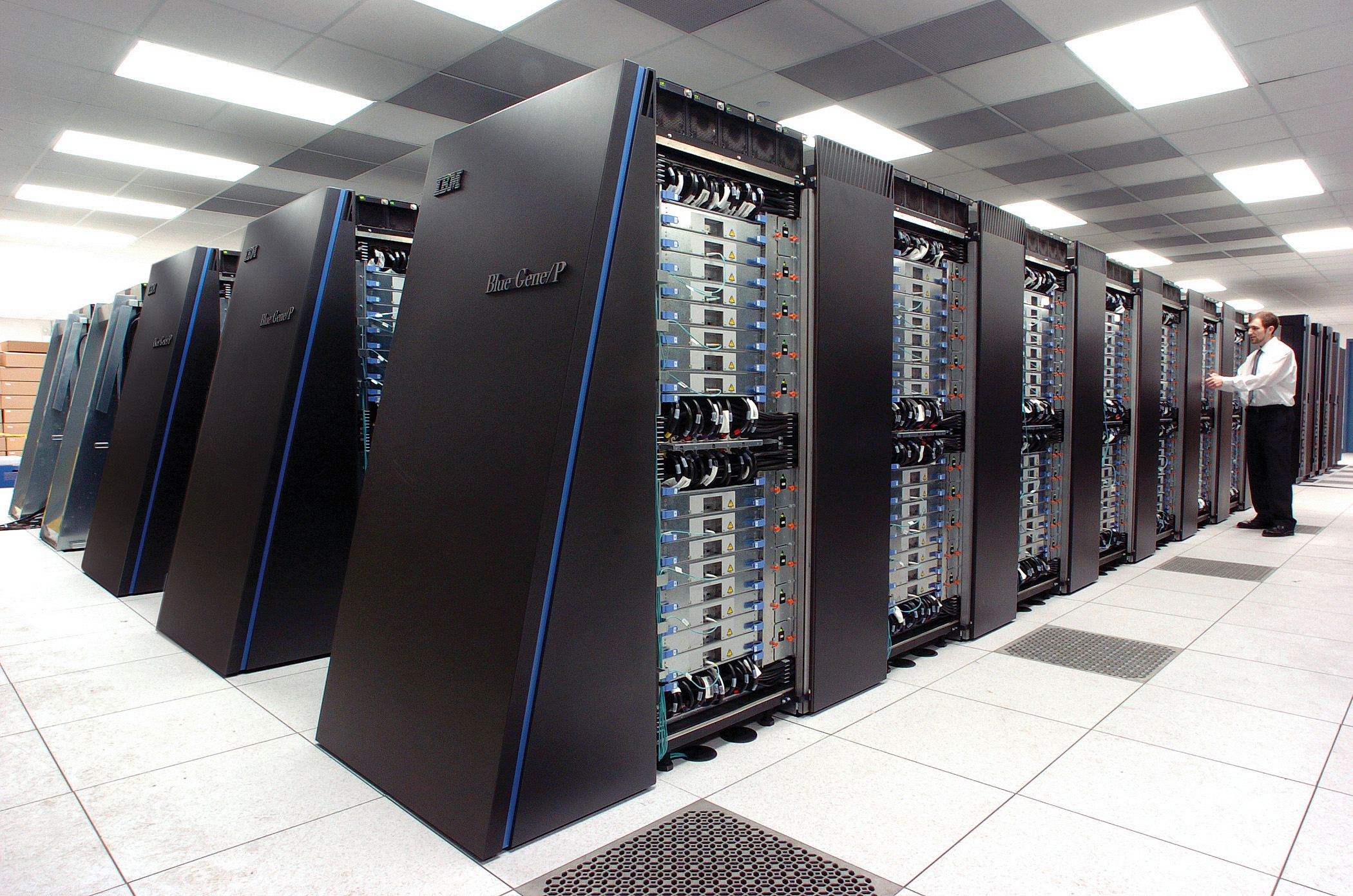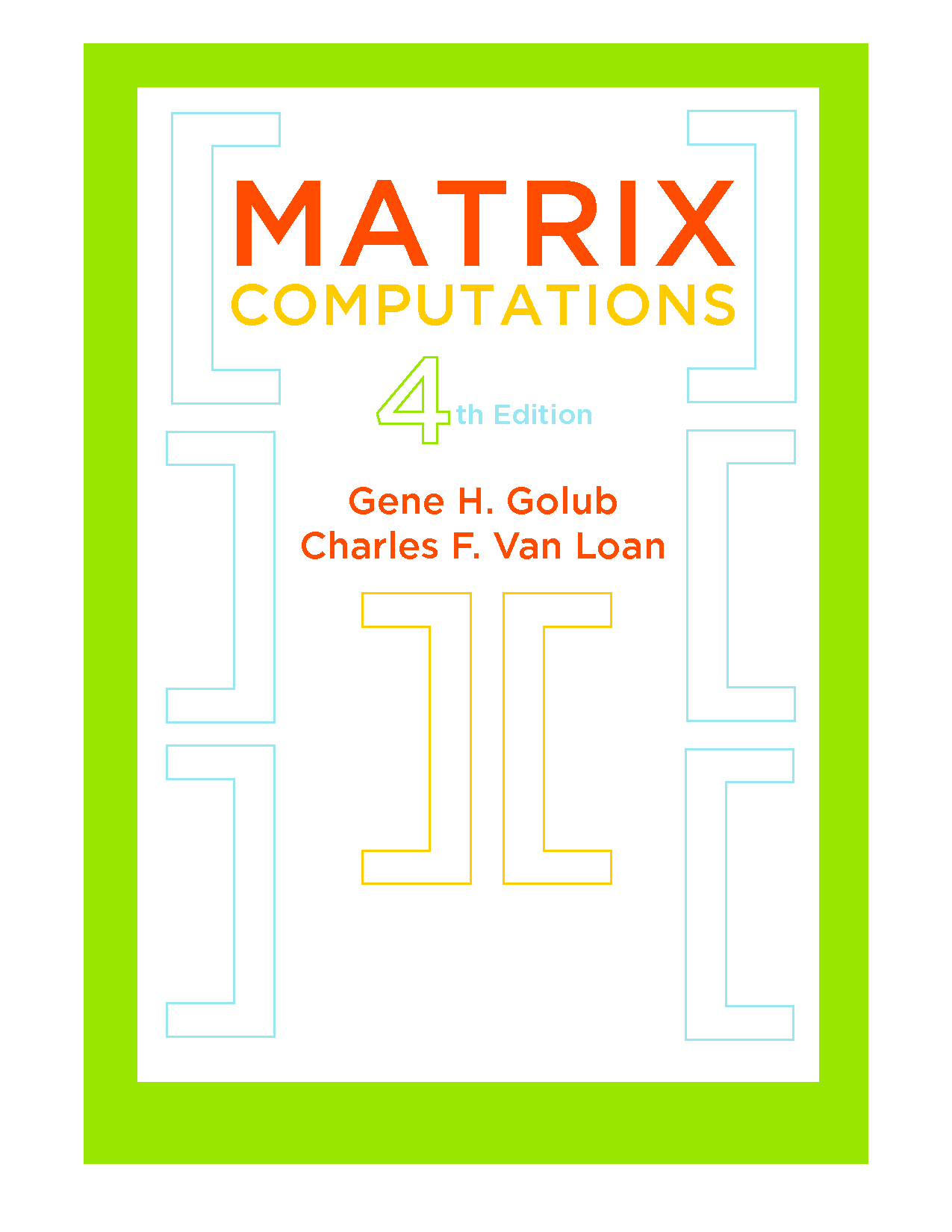#### Numerical Analysis: Linear and Nonlinear Problems (CS 4220/5223 / MATH 4260)

Olin 255. MWF 11:20-12:10.

Fundamentals of numerical linear algebra (linear systems, eigenvalues and SVDs), on which we’ll build iterative methods for nonlinear systems and multivariate optimization. Understanding advantages, disadvantages, and practical limits of numerical methods. Programming required.#### Applications of Parallel Computers (CS 5220)

Overview of high performance scientific computing. Basics of modern parallel machine design, parallel programming patterns, and applications. Programming projects will likely inspire creative cursing, mostly due to the frustrations of parallel profiling and debugging.#### Matrix Computations (CS 6210)

Numerical linear algebra. Basic theory, efficient algorithms, and enough error analysis to avoid numerical embarrassment (we hope)! Direct and iterative methods for linear systems, least squares, and eigenvalues.#### Numerical Methods for Data Science (CS 6241)

Discussion of numerical methods in the context of machine learning and data analysis problems. We will discuss sparsity, rank structure, and spectral behavior of underlying linear algebra problems; convergence behavior and implicit regularization for standard solvers; and comparisons between numerical methods in data analysis and those used in physical simulations.#### Introduction to Scientific Computing (CS 3220)

No longer offered; see CS 4210 and CS 4220.

Overview of scientific computing in MATLAB, from a user perspective. Error analysis basics, linear systems and least squares, nonlinear equations, basic optimization, numerical differentiation and quadrature, ODEs, and applications.#### SCAN Seminar (CS/MATH 7290)

Ongoing. M 1:30-2:20.

The Scientific Computing and Numerics seminar series focuses on various methods in scientific computing, the analysis of convergence properties and computational efficiency, and their adaptation to specific applications.#### Projects (CS 999)

See my list of projects!

If nothing on the list appeals but you think you’d like to work with me on something, come knock on my door. I don’t bite.#### Numerical Methods for Data Science (SJTU CS 259)

Survey course of numerical methods prominent in modern data analysis and machine learning. Building on basic methods of optimization and numerical linear algebra, the course will explore the role of numerical methods for treating several classes of data analysis problems, including low rank factorizations and completion of matrix data; function approximation by kernel methods; and analysis of data on graphs.

See here for older courses.